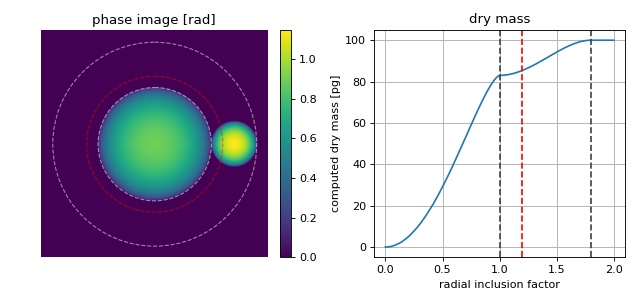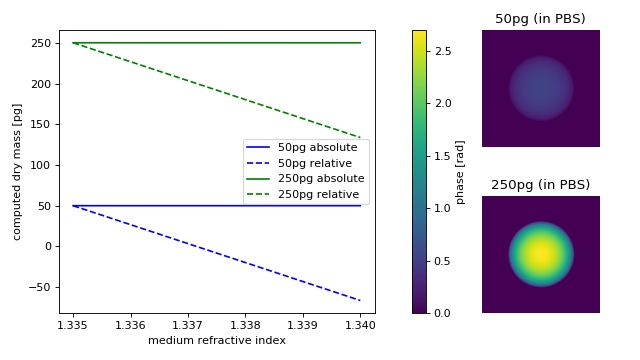Code examples¶

Dry mass computation with radial inclusion factor¶

This examples illustrates the usage of the “radial inclusion factor” which is defined in the configuration section “sphere” and used in drymass.anasphere.relative_dry_mass() with the keyword argument rad_fact.

The phase image is computed from two spheres whose dry masses add up to 100pg with the larger sphere having a dry mass of 83pg. The larger sphere is located at the center of the image which is also used as the origin for dry mass computation. The radius of the larger sphere is known (10µm). Thus, the corresponding radius (inner circle) corresponds to a radial inclusion factor of 1. In DryMass, the default radial inclusion factor is set to 1.2 (red). In some cases, this inclusion factor must be increased or decreased depending on whether additional information (the smaller sphere) should be included in the dry mass computation or not.Comparison of relative and absolute dry mass¶

Relative dry mass is the dry mass computed relative to the surrounding medium. If the refractive index of the surrounding medium does not match that of the intracellular fluid (approximately 1.335), then the relative dry mass underestimates the actual dry mass. For a spherical cell, the absolute (corrected) dry mass can be computed as described in the theory section on dry mass computation.

This examples compares the relative dry mass (drymass.ansphere.relative_dry_mass()) to the absolute dry mass corrected for a spherical phase object (drymass.ansphere.absolute_dry_mass_sphere()). From simulated phase images (projection approach, wavelength 550nm) of two cell-like spheres with a radius of 10µm and dry masses of 50pg (n≈1.337) and 250pg (n≈1.346), the absolute and relative dry masses are computed with varying refractive index of the medium.

At the refractive index of phosphate buffered saline (PBS), absolute and relative dry mass are equivalent. As the refractive index of the medium increases, the relative drymass decreases linearly (independent of dry mass), underestimating the actual dry mass.mass_relative_vs_absolute.py

 1 2 3 4 5 6 7 8 9 10 11 12 13 14 15 16 17 18 19 20 21 22 23 24 25 26 27 28 29 30 31 32 33 34 35 36 37 38 39 40 41 42 43 44 45 46 47 48 49 50 51 52 53 54 55 56 57 58 59 60 61 62 63 64 65 66 67 68 69 70 71 72 73 74 75 76 77 78 79 80 81 82 83 84 85 86 87 88 89 90 91 92 93 94 95 from drymass.anasphere import absolute_dry_mass_sphere, relative_dry_mass import matplotlib import matplotlib.pylab as plt import numpy as np import qpsphere # refraction increment alpha = .18 # [mL/g] # general simulation parameters model = "projection" wavelength = 500e-9 # [m] pixel_size = 1.8e-7 # [m] grid_size = (200, 200) # [px] # sphere parameters radius = 10 # [µm] center = (100, 100) # [px] dry_masses = [50, 250] # [pg] medium_indices = np.linspace(1.335, 1.34, 5) qpi_pbs = {} m_abs = {} m_rel = {} phase_data = np.zeros(grid_size, dtype=float) for m in dry_masses: # initiate results list m_abs[m] = [] m_rel[m] = [] # compute refractive index from dry mass r_m = radius * 1e-6 alpha_m3g = alpha * 1e-6 m_g = m * 1e-12 n = 1.335 + 3 * alpha_m3g * m_g / (4 * np.pi * (r_m**3)) for medium_index in medium_indices: # generate example dataset qpi = qpsphere.simulate(radius=r_m, sphere_index=n, medium_index=medium_index, wavelength=wavelength, pixel_size=pixel_size, model=model, grid_size=grid_size, center=center) # absolute dry mass ma = absolute_dry_mass_sphere(qpi=qpi, radius=r_m, center=center, alpha=alpha, rad_fact=1.2) m_abs[m].append(ma * 1e12) # relative dry mass mr = relative_dry_mass(qpi=qpi, radius=r_m, center=center, alpha=alpha, rad_fact=1.2) m_rel[m].append(mr * 1e12) if medium_index == 1.335: qpi_pbs[m] = qpi # plot results fig = plt.figure(figsize=(8, 4.5)) matplotlib.rcParams["image.interpolation"] = "bicubic" # phase images kw = {"vmax": qpi_pbs[dry_masses].pha.max(), "vmin": qpi_pbs[dry_masses].pha.min()} ax1 = plt.subplot2grid((2, 3), (0, 2)) ax1.set_title("{}pg (in PBS)".format(dry_masses)) ax1.axis("off") map1 = ax1.imshow(qpi_pbs[dry_masses].pha, **kw) ax2 = plt.subplot2grid((2, 3), (1, 2)) ax2.set_title("{}pg (in PBS)".format(dry_masses)) ax2.axis("off") ax2.imshow(qpi_pbs[dry_masses].pha, **kw) # overview plot ax3 = plt.subplot2grid((2, 3), (0, 0), colspan=2, rowspan=2) ax3.set_xlabel("medium refractive index") ax3.set_ylabel("computed dry mass [pg]") for m, c in zip(dry_masses, ["blue", "green"]): ax3.plot(medium_indices, m_abs[m], ls="solid", color=c, label="{}pg absolute".format(m)) ax3.plot(medium_indices, m_rel[m], ls="dashed", color=c, label="{}pg relative".format(m)) ax3.legend() plt.colorbar(map1, ax=ax3, fraction=.048, pad=0.1, label="phase [rad]") plt.tight_layout() plt.subplots_adjust(wspace=.14) plt.show()